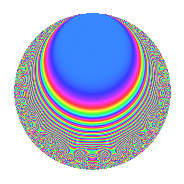# Properties

 Label 105.3.fLevel $105$ Weight $3$ Character orbit 105.f Rep. character $\chi_{105}(29,\cdot)$ Character field $\Q$ Dimension $24$ Newform subspaces $1$ Sturm bound $48$ Trace bound $0$

# Related objects

## Defining parameters

 Level: $$N$$ $$=$$ $$105 = 3 \cdot 5 \cdot 7$$ Weight: $$k$$ $$=$$ $$3$$ Character orbit: $$[\chi]$$ $$=$$ 105.f (of order $$2$$ and degree $$1$$) Character conductor: $$\operatorname{cond}(\chi)$$ $$=$$ $$15$$ Character field: $$\Q$$ Newform subspaces: $$1$$ Sturm bound: $$48$$ Trace bound: $$0$$

## Dimensions

The following table gives the dimensions of various subspaces of $$M_{3}(105, [\chi])$$.

Total New Old
Modular forms 36 24 12
Cusp forms 28 24 4
Eisenstein series 8 0 8

## Trace form

 $$24q + 52q^{4} - 22q^{9} + O(q^{10})$$ $$24q + 52q^{4} - 22q^{9} - 24q^{10} + 26q^{15} + 4q^{16} + 72q^{19} + 14q^{21} - 156q^{24} - 64q^{25} - 32q^{30} - 40q^{31} - 144q^{34} + 36q^{36} + 62q^{39} - 40q^{40} + 120q^{45} - 104q^{46} - 168q^{49} + 70q^{51} + 60q^{54} - 16q^{55} - 348q^{60} + 432q^{61} - 364q^{64} + 284q^{66} + 404q^{69} + 140q^{70} + 204q^{75} + 152q^{76} + 108q^{79} - 158q^{81} + 112q^{84} + 196q^{85} - 152q^{90} - 84q^{91} + 808q^{94} - 516q^{96} + 582q^{99} + O(q^{100})$$

## Decomposition of $$S_{3}^{\mathrm{new}}(105, [\chi])$$ into newform subspaces

Label Dim. $$A$$ Field CM Traces $q$-expansion
$$a_2$$ $$a_3$$ $$a_5$$ $$a_7$$
105.3.f.a $$24$$ $$2.861$$ None $$0$$ $$0$$ $$0$$ $$0$$

## Decomposition of $$S_{3}^{\mathrm{old}}(105, [\chi])$$ into lower level spaces

$$S_{3}^{\mathrm{old}}(105, [\chi]) \cong$$ $$S_{3}^{\mathrm{new}}(15, [\chi])$$$$^{\oplus 2}$$